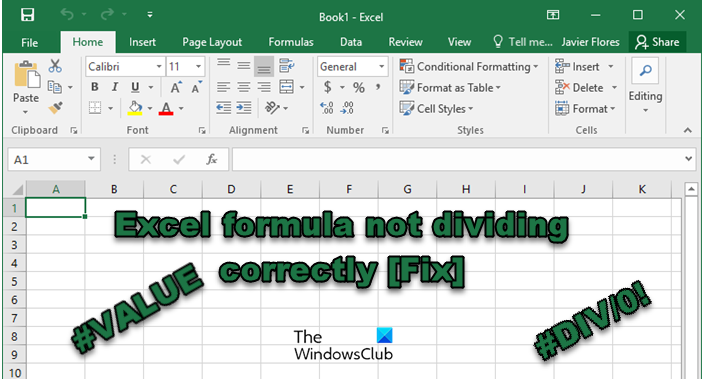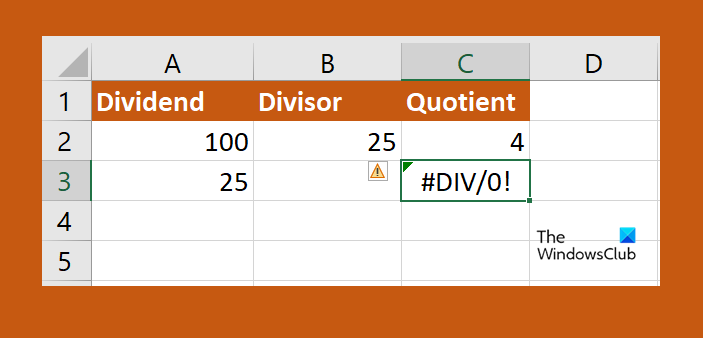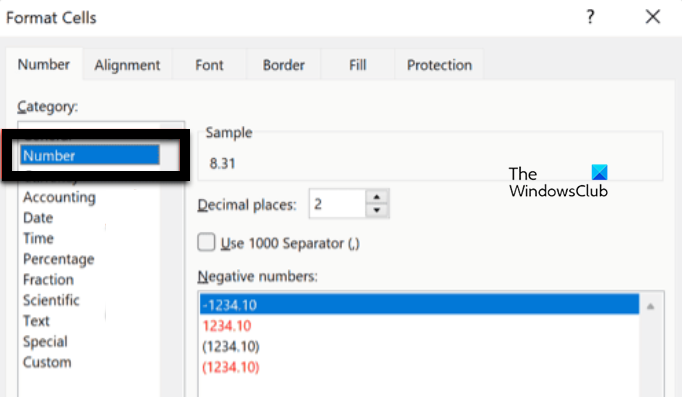Saturday, September 30, 2023
HomeWINDOWSExcel formula not dividing correctly

# Excel formula not dividing correctly [Fix]

-

Excel is arguably one of the best spreadsheet applications on the planet, but this doesn’t mean that it is perfect. Some users complain that the Excel formula is not dividing correctly, In this post, we will talk about this issue and see what you can do if you see #DIV/0! error or some other error when dividing in Excel.### Why is Excel not calculating division correctly?

Excel formulas are not calculating properly as there must have been some mistake you made when entering the numbers in the cell. Or if the cells are not formatted correctly. In that case, we need to make amendments to the settings and make sure that all the values entered in the cells are legit.

## Fix Excel formula not dividing correctly

If the Excel formula is not dividing correctly, follow the solutions mentioned below to resolve the issue.

1. Make sure you are not dividing by Zero
2. Resolve #DIV/0! Error
3. Increase the cell width to know the what number you have entered
4. Formal the cells
5. Disable Manual Calculation

Let us talk about them in detail.

#### 1] Make sure you are not dividing by Zero

First of all, we need to check if you are dividing the number with zero, that is, the denominator has zero. If you have studied mathematics, you know no number can be divided by zero. Hence, check if you are doing the same, and make the required amendments.

#### 2] Resolve #DIV/0! ErrorIt is pretty likely that when performing operations on a large number of data, you left some zero. In that case, we need to replace #DIV/0! errors in your spreadsheet. Follow the steps given below to do the same.

1. Click on the top-most cell of the column where you want the division to happen and enter =IF(.
2. Now, click on the cell that contains the denominators and enter ‘=0, “N/A”,’.
3. Click on the cell you want to divide, type /, and click on the cell you are dividing by.
4. Enter a closed bracket and then click on the Enter button.
5. Now, you can click on the little green mark and extend it to the last cell where you want the formula to be applied.

If a number is divided by zero, it will say NA. And hopefully, this will do the job for you.

#### 3] Increase the cell width to know the what number you have entered

If you think that the division result is not correct, you should increase the width of the cell. That’s because, if you enter a large number, it is very likely that you are unable to see the number that you have entered in the cell due to it not being large enough. In that case, all there is to do is click on the boundary of the column and extend it. Finally, you will have knowledge of the numbers you have entered and can make amendments as required.

#### 4] Format the cellsIn case, Excel says #VALUE in a cell, it is formatted properly. This issue occurs when one or multiple cells are set to Numbers but their decimal is not configured correctly. We need to make the required changes, follow the steps given below to do the same.

1. In Excel, go to Home to check the format of the cell.
2. Then, select the cells you want to reconfigure using the Shift button.
3. Right-click on them and select Format Cells.
4. Then select Number and then configure decimal correctly.

Hopefully, you are able to resolve the issue using this solution.

#### 5] Disable Manual Calculation

In Manual Calculation, the user needs to force it. This can work when you are dealing with a small spreadsheet, but when you are working with an enormous amount of data, this won’t work. That is why, we need to disable the settings to resolve the issue. Follow the steps given below to do the same.

1. In Excel, go to File.
2. Now, go to Options > Formulas.
3. Finally, select Automatic.

We hope that you are able to resolve the issue using the solutions mentioned in this post.

### How do I fix the division formula in Excel?

If the Division formula is not calculating correctly, you need to execute the solutions mentioned in this post to resolve the issue. We recommend you start with the first solution and then move your way down. You will surely be able to resolve the issue.

Also Read: Excel not opening on Windows computer.